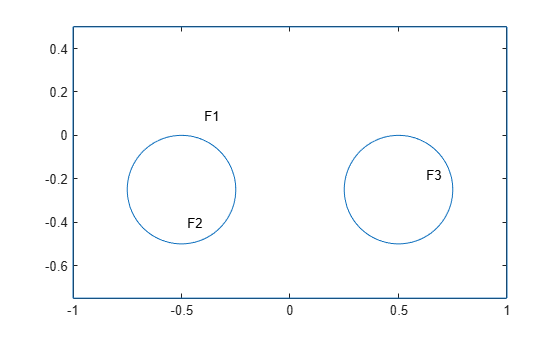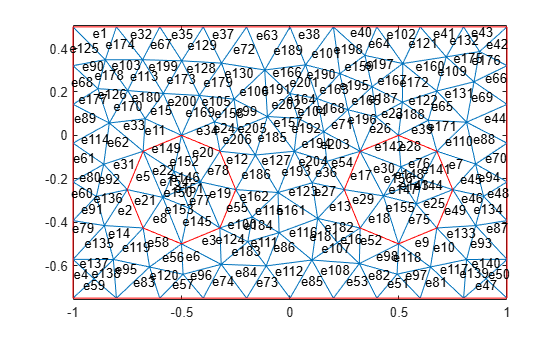pdesdt

Indices of subset of mesh elements belonging to specified faces of 2-D geometry

pdesdt is not recommended. Use findElements instead.

Description

example

i = pdesdt(t,FaceID) returns the indices of the mesh elements of the [p,e,t] mesh that belong to the set of faces listed in FaceID.

i = pdesdt(t) assumes that FaceID is a list of all faces of a 2-D geometry.

Examples

collapse all

Find the indices of the mesh elements belonging to the specified faces.

Define two circles and a rectangle and place these in one matrix.

R1 = [3,4,-1,1,1,-1,0.5,0.5,-0.75,-0.75]';
C1 = [1,-0.5,-0.25,0.25]';
C2 = [1,0.5,-0.25,0.25]';
C1 = [C1;zeros(length(R1) - length(C1),1)];
C2 = [C2;zeros(length(R1) - length(C2),1)];
gd = [R1,C1,C2];

Create a set formula that adds the circles to the rectangle.

sf = 'R1+C1+C2';

Create and plot the geometry.

ns = char('R1','C1','C2');
ns = ns';
gd = decsg(gd,sf,ns);
pdegplot(gd,'FaceLabels','on')Create a mesh.

[p,e,t] = initmesh(gd);

Plot the mesh with the element labels.

pdemesh(p,e,t,'ElementLabels','on')Find the indices of the mesh elements that belong to face 2. Display the result as a column vector.

i = pdesdt(t,2);
i.'
ans = 16×1

5
8
19
20
21
22
75
76
139
140
⋮

Input Arguments

collapse all

Mesh elements, specified as a 4-by-Nt matrix of triangles, where Nt is the number of triangles in the mesh. For details on the mesh data representation, see initmesh.

Data Types: double

Face IDs, specified as a vector of integers.

Data Types: double

Output Arguments

collapse all

Mesh elements belonging to the specified faces, returned as a vector of integers.